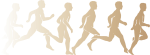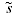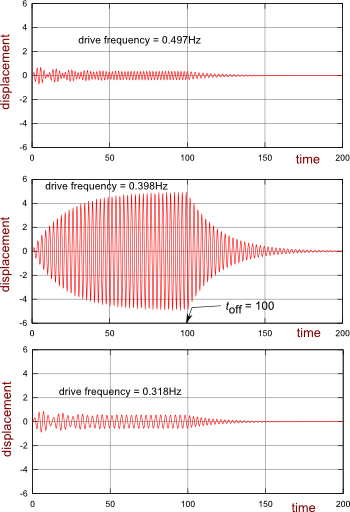There are all sorts of resonances around us, in the world, in our culture, and in our technology. A tidal resonance causes the 55 foot tides in the Bay of Fundy. Mechanical and acoustical resonances and their control are at the center of practically every musical instrument that ever existed. Even our voices and speech are based on controlling the resonances in our throat and mouth. Technology is also a heavy user of resonance. All clocks, radios, televisions, and gps navigating systems use electronic resonators at their very core. Doctors use magnetic resonance imaging or MRI to sense the resonances in atomic nuclei to map the insides of their patients. In spite of the great diversity of resonators, they all share many common properties. In this blog, we will delve into their various aspects. It is hoped that this will serve both the students and professionals who would like to understand more about resonators. I hope all will enjoy the animations.

Origins of Newton's laws of motion

History of mechanical clocks with animations
Understanding a mechanical clock with animations
includes pendulum, balance wheel, and quartz clocks

## Friday, June 22, 2012

### Build up and decay of the envelope

 All postings by author previous: 3.9 Summing impulses with calculus up: Contents details:Supplement next: Various Q's

Build up and decay of the envelopeFig. 20. This graph more clearly shows the exponential nature of the build up and decay of the resonance. The exponential envelope is shown in green. This graph is similar to that of Fig. 18 of the previous posting except that the time period is longer, the Q is higher (equals 25), and only a drive frequency of 0.398Hz is shown. The driver is turned on at t = 0 and turned off at t = 100sec.On resonance

While Figure 19 shows the relative phase of the driver and oscillations of the mass, the exponential nature of the envelope is not so clear there. The next figure (Fig. 20, at the right) is optimized to show this exponential nature more clearly. The first part of this graph (the build up part) was made using (96) with ω set equal to ωdecay.

The second part (the decay part) was made using (81) and adjusting the amplitude A and phase φ of the decaying oscillations so that the position x and the velocity v of the mass were the same two values immediately after the drive pulse was turned off (immediately after 100sec on the graph) as they were immediately before the drive pulse was turned off (an instant before 100 seconds on the graph). That is to say:

 We adjusted A and φ in the decay equation (81) after 100s so that: x (just after 100s) = x (just before 100s) (99) v (just after 100s) = v (just before 100s)

The math for this adjustment is explained in the "off resonance" section below, which actually applies to both on-resonance and off-resonance conditions.

If the drive frequency equals the resonant frequency and Q >> 1, the amplitude (as shown in green in Fig. 20) is approximately given by (100):

The maximum amplitude A in (100) is given by A = f0Q/2 , which is derived in detail in the supplemental page.

The first part of (100) can be derived from (96) with the requirement that ω = ωdecay and that the Q is large, i.e. Q >> 1. The second part of (100) is just the free decay of a resonator as discussed many places in this blog, for example in the damping section above.

Off resonance

Because the off-resonance behavior of a resonator is fairly complicated we shall only set up the equations that will allow us to graph it, without arriving at a nice compact equation like (100) above for the on-resonance behavior. As in the on-resonance case, the challenge for plotting the build up and decay of a sinusoidal pulse exciting a resonator is matching the decay equation to the build up equation at the time the sinusoidal excitation pulse turns off.

Equation (98) above gives the build up with time.

The decay equation, Equation (1), is much simpler and is of the form:

where we have replaced t in (1) with t − toff to allow for the decay starting at t = toff instead of t = 0. We have also added an adjustable phase φ as needed to match the decay with the excitation at t = toff .

Repeating the requirements listed in (99) above: we need to adjust the amplitude A and the phase φ so that both the displacement x and the velocity v = dx/dt  of (101) equal that of (98) at the time t = toff .

We now change x(t) in (101) into the complex forms. We do the same for the mass's velocity v = dx/dt:

The tilda above x, v, A and s in (102) is optional and serves to remind us that that they are complex. The complex amplitudeand exponential multipliercontain the phase shifts φ and arg() , i.e.:

where A and s without the tilda are meant to be the magnitude of those quantities in this particular derivation.

The real part of (102) taken at t = toff  is:.     (105)

We need to remember that in this equation s means the magnitude of the
complex.Fig. 21. Motion of the mass in response to a burst of sinusoidal excitation of various frequencies. The resonant frequency of the mass/spring resonator is 0.398Hz and its Q is 25. The drive excitation equals 0.1 sin ωt for times 0t100sec and the drive equals 0 for times t>100sec. When the drive frequency equals the resonant frequency (the middle graph), the build up and decay of oscillations follow the simple exponential equation (100). At other frequencies, we see less of a build up which is more complex and faster to assume a steady state level. The decay after the drive pulse is finished is a simple exponential decay in all three cases with the same time constant τ and the decaying oscillations are all at the frequency ωdecay .

Dividing v by x gives:

where x and v are the actual displacement and displacement velocity of the oscillator at the instant the driving pulse is turned off (at t = toff ).

We solve for φ:

Reviewing (103) we see that the real part ofequals −1/τ and the imaginary part equals ωdecay. The cosine of the argument of any complex number equals its real part over its magnitude. Similarly, the sine of the argument of a complex number equals its imaginary part over its magnitude. Thus we can write for:

which makes (106) become:

Using (107) we can use the position and velocity of the mass just before toff to calculate the correct phase φ  to use in (101) for the graph of x(t) after t = toff . The amplitude of the decay can be had by solving the first equation in (105) for A:

At the right in Fig. 21, we show the filling and decay curves for bursts of several frequencies. We see that the off-resonance build up is fairly complicated, similar to that seen off-resonance in the animated figures 15a, 15e, 16c and 16d of the previous postings. At the end of the previous posting, we discussed the initial phase overshoot which causes the initial amplitude overshoot in the off resonance curves.

In Fig. 21, we actually see several oscillations in amplitude in the off-resonance curves before they settle to a steady state value of the amplitude and phase shown by Fig. 9 of an earlier posting. These initial oscillations in amplitude are the result of a beating between the drive frequency and the resonance frequency of the resonator. To be specific, we expect a beat frequency for the top graph of fbeat = 0.497− 0.398 = 0.099Hz . This beat frequency would have a period of Tbeat = 1/fbeat = 1/0.099 = 10.1sec, which is the beat period seen in the left side of the top graph. This beating seems to die off with decay time τ given by (24), the same τ that governs the build up and decay of the on-resonance amplitude shown in Fig. 20 and Equation (100) above.

These graphs illustrate that the earlier smooth graphs of amplitude and phase versus the drive frequency represent only the steady-state behavior of a resonator, after the transients have settled down.

 All postings by author previous: 3.9 Summing impulses with calculus up: Contents details:Supplement next: Various Q's